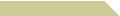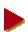HOME COURSES NOTES EXAMS RÉSUMÉ

### TESTING PRIMES TO NINE QUADRILLION

Enter a number N >>

##Known Composites, Unknown Factors

Two properties are common to all prime numbers p and positive integers a < p. First is the fact that ap−1 % p = 1, which is refered to as the Fermat's little theorem (FLT). The second, if a2 % p = 1, then either a % p = p−1 or a % p = 1. So, suppose we know that 1234 % 35 = 9, instead of 1. Then we may conclude that 35 is composite, with or without the knowledge that 35 = 5 × 7. If that were not enough, another evidence that 35 cannot possibly be a prime is the truth that 62 % 35 = 1, where 6 is neither 1 nor 35−1.

Now given a large odd integer N, we compute the odd number d for which (N−1) ÷ d = 2e and then look at the following sequence of e+1 numbers,

ad, a2d, a22d, a23d, … , a2ed = aN−1

all reduced % N. Note that each term is succeeded by its square. Hence if we spot a 1 that is preceded by neither 1 nor N−1, then N is composite. Or, if the last number is not 1, then N is composite by FLT. This algorithm is what we call the Miller-Rabin test (MRT).

The bad news is MRT seems to catch only "some" composites, i.e., those which fail the test. The good news is, the composites that do pass MRT are quite rare. Moreover, why not we run the test with different values of a, further reducing the number of composites which possibly survive the multiple bases. And if these few so-called "strong pseudoprimes" have been predetermined within a given interval, then MRT becomes an absolute primality test.

Note that we are not concerned with finding the factors of a composite N. See our trial division page if you are looking for a factoring algorithm.

##This Implementation

We initially check if N is divisible a small prime below 100 before we proceed with MRT. Then N is subjected to four rounds of MRT where the bases are chosen to be 2, 3, 5, and 7. If to any base N is recognized to be a composite, we immediately terminate the algorithm. Otherwise, N is either a prime or a strong pseudoprime.

As for now, JavaScript allows a maximum size of 53 bits for an integer, where 253 = 9,007,199,254,740,992. In this range, there are exactly 580 strong pseudoprimes to those four bases—a mere two in every 31 trillion! A quick scan through our pseudoprime database then reveals whether or not N is a genuine prime.

Feel free to save or modify a copy of this file for your own use.

It won't be a surprise if someone somewhere has already found a way to circumvent the 53-bit JavaScript constraint. Let me know.

##Do Not Repeat These Mistakes

Computing modular exponentiation involves powers of 2, so it was tempting to use bitwise operators such as the left and right binary shifts. In JavaScript, however, such an operation will transform all operands to 32-bit integers!

Also, modular exponentiation is typically performed via successive squaring in order to keep the intermediate outputs smaller than N. Even so, when N > 226.5 squaring a number may violate the 53-bit limit. In such a case we resort to long-multiplication by the right-to-left binary digits. In other words, successive squaring will have to be downgraded to successive doubling! A similar caution applies to large modular addition.

It is possible to scan an array contents, such as the pseudoprimes we store, using the indexOf function, which returns the index of an element or −1 otherwise. We discovered that indexOf was originally written for string variables but was only recently extended to arrays. Beware that older browsers may not support this new feature unless we insert the extension subroutine. But again, all we really need is to know the existence, not the index.